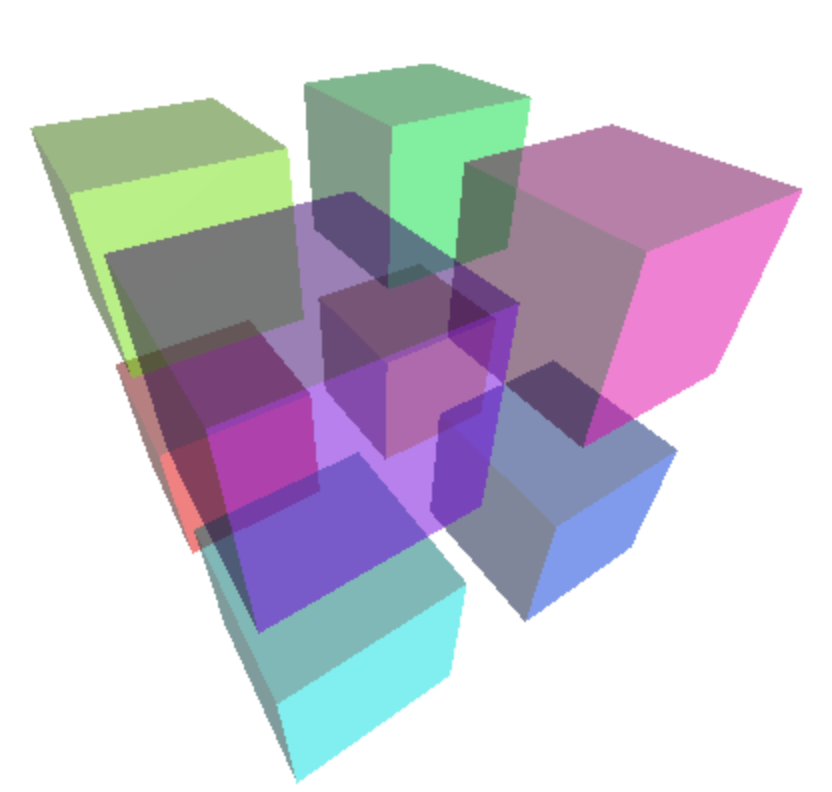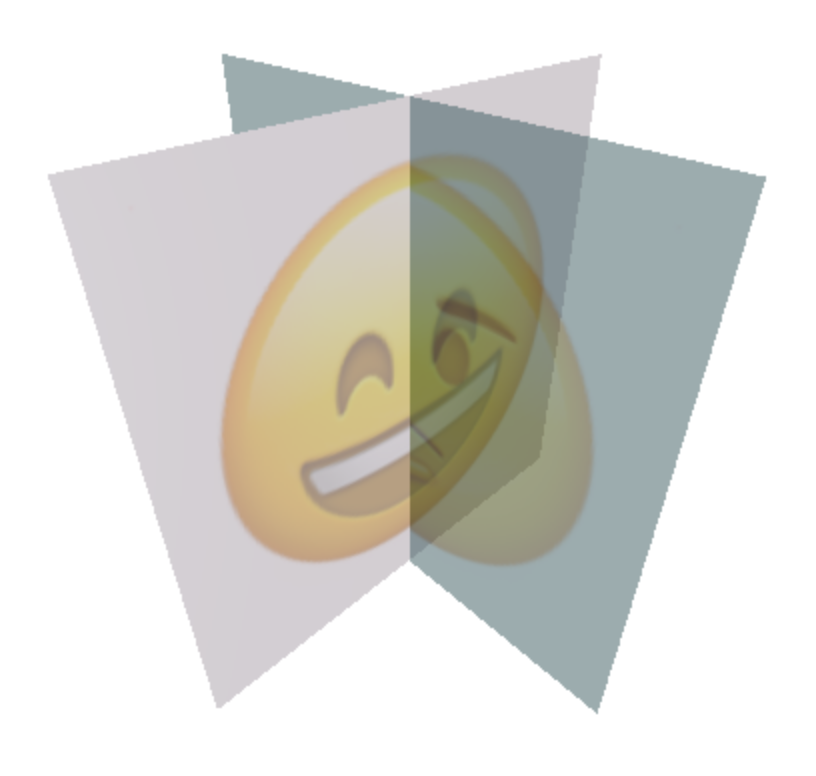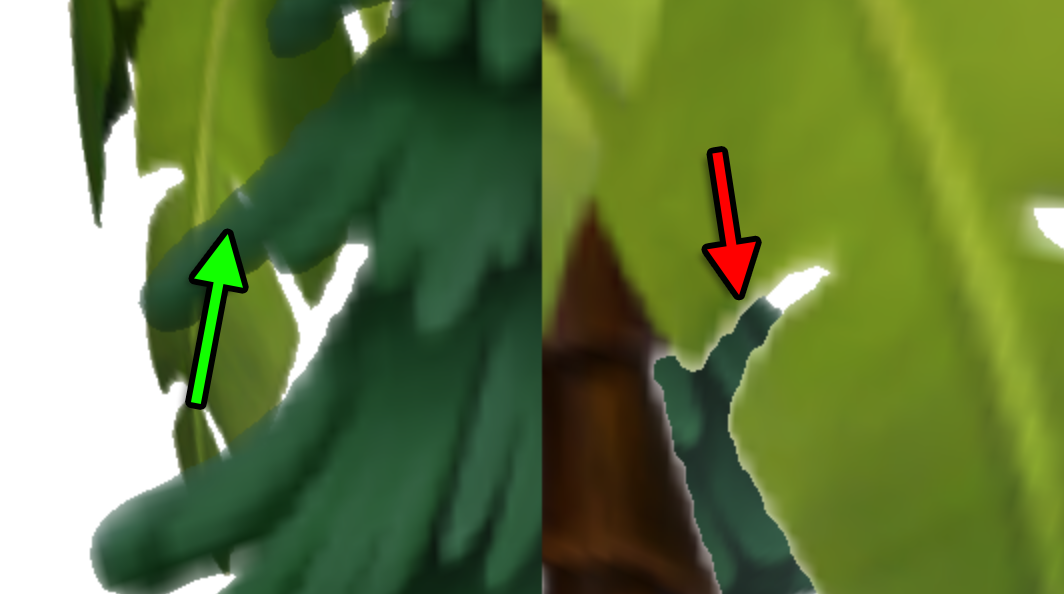# threejsfundamentals.org

Fix, Fork, Contribute

# Three.js Transparency

Transparency in three.js is both easy and hard.

First we'll go over the easy part. Let's make a scene with 8 cubes placed in a 2x2x2 grid.

We'll start with the example from the article on rendering on demand which had 3 cubes and modify it to have 8. First let's change our `makeInstance` function to take an x, y, and z

```-function makeInstance(geometry, color) {
+function makeInstance(geometry, color, x, y, z) {
const material = new THREE.MeshPhongMaterial({color});

const cube = new THREE.Mesh(geometry, material);

-  cube.position.x = x;
+  cube.position.set(x, y, z);

return cube;
}
```

Then we can create 8 cubes

```+function hsl(h, s, l) {
+  return (new THREE.Color()).setHSL(h, s, l);
+}

-makeInstance(geometry, 0x44aa88,  0);
-makeInstance(geometry, 0x8844aa, -2);
-makeInstance(geometry, 0xaa8844,  2);

+{
+  const d = 0.8;
+  makeInstance(geometry, hsl(0 / 8, 1, .5), -d, -d, -d);
+  makeInstance(geometry, hsl(1 / 8, 1, .5),  d, -d, -d);
+  makeInstance(geometry, hsl(2 / 8, 1, .5), -d,  d, -d);
+  makeInstance(geometry, hsl(3 / 8, 1, .5),  d,  d, -d);
+  makeInstance(geometry, hsl(4 / 8, 1, .5), -d, -d,  d);
+  makeInstance(geometry, hsl(5 / 8, 1, .5),  d, -d,  d);
+  makeInstance(geometry, hsl(6 / 8, 1, .5), -d,  d,  d);
+  makeInstance(geometry, hsl(7 / 8, 1, .5),  d,  d,  d);
+}
```

```const fov = 75;
const aspect = 2;  // the canvas default
const near = 0.1;
-const far = 5;
+const far = 25;
const camera = new THREE.PerspectiveCamera(fov, aspect, near, far);
-camera.position.z = 4;
+camera.position.z = 2;
```

Set the background to white

```const scene = new THREE.Scene();
+scene.background = new THREE.Color('white');
```

And added a second light so all sides of the cubes get some lighting.

```-{
const color = 0xFFFFFF;
const intensity = 1;
const light = new THREE.DirectionalLight(color, intensity);
-  light.position.set(-1, 2, 4);
+  light.position.set(...pos);
}
```

To make the cubes transparent we just need to set the `transparent` flag and to set an `opacity` level with 1 being completely opaque and 0 being completely transparent.

```function makeInstance(geometry, color, x, y, z) {
-  const material = new THREE.MeshPhongMaterial({color});
+  const material = new THREE.MeshPhongMaterial({
+    color,
+    opacity: 0.5,
+    transparent: true,
+  });

const cube = new THREE.Mesh(geometry, material);

cube.position.set(x, y, z);

return cube;
}
```

and with that we get 8 transparent cubes

Drag on the example to rotate the view.

So it seems easy but ... look closer. The cubes are missing their backs.no backs

We learned about the `side` material property in the article on materials. So, let's set it to `THREE.DoubleSide` to get both sides of each cube to be drawn.

```const material = new THREE.MeshPhongMaterial({
color,
opacity: 0.5,
transparent: true,
+  side: THREE.DoubleSide,
});
```

And we get

Give it a spin. It kind of looks like it's working as we can see backs except on closer inspection sometimes we can't.the left back face of each cube is missing

This happens because of the way 3D objects are generally drawn. For each geometry each triangle is drawn one at a time. When each pixel of the triangle is drawn 2 things are recorded. One, the color for that pixel and two, the depth of that pixel. When the next triangle is drawn, for each pixel if the depth is deeper than the previously recorded depth no pixel is drawn.

This works great for opaque things but it fails for transparent things.

The solution is to sort transparent things and draw the stuff in back before drawing the stuff in front. THREE.js does this for objects like `Mesh` otherwise the very first example would have failed between cubes with some cubes blocking out others. Unfortunately for individual triangles shorting would be extremely slow.

The cube has 12 triangles, 2 for each face, and the order they are drawn is the same order they are built in the geometry so depending on which direction we are looking the triangles closer to the camera might get drawn first. In that case the triangles in the back aren't drawn. This is why sometimes we don't see the backs.

For a convex object like a sphere or a cube one kind of solution is to add every cube to the scene twice. Once with a material that draws only the back facing triangles and another with a material that only draws the front facing triangles.

```function makeInstance(geometry, color, x, y, z) {
+  [THREE.BackSide, THREE.FrontSide].forEach((side) => {
const material = new THREE.MeshPhongMaterial({
color,
opacity: 0.5,
transparent: true,
+      side,
});

const cube = new THREE.Mesh(geometry, material);

cube.position.set(x, y, z);
+  });
}
```

Any with that it seems to work.

It assumes that the three.js's sorting is stable. Meaning that because we added the `side: THREE.BackSide` mesh first and because it's at the exact same position that it will be drawn before the `side: THREE.FrontSide` mesh.

Let's make 2 intersecting planes (after deleting all the code related to cubes). We'll add a texture to each plane.

```const planeWidth = 1;
const planeHeight = 1;
const geometry = new THREE.PlaneGeometry(planeWidth, planeHeight);

function makeInstance(geometry, color, rotY, url) {
const material = new THREE.MeshPhongMaterial({
color,
map: texture,
opacity: 0.5,
transparent: true,
side: THREE.DoubleSide,
});

const mesh = new THREE.Mesh(geometry, material);

mesh.rotation.y = rotY;
}

makeInstance(geometry, 'pink',       0,             'resources/images/happyface.png');
makeInstance(geometry, 'lightblue',  Math.PI * 0.5, 'resources/images/hmmmface.png');
```

This time we can use `side: THREE.DoubleSide` since we can only ever see one side of a plane at a time. Also note we pass our `render` function to the texture loading function so that when the texture finishes loading we re-render the scene. This is because this sample is rendering on demand instead of rendering continuously.

And again we see a similar issue.half a face is missing

The solution here is to manually split the each pane into 2 panes so that there really is no intersection.

```function makeInstance(geometry, color, rotY, url) {
+  const base = new THREE.Object3D();
+  base.rotation.y = rotY;

+  [-1, 1].forEach((x) => {
+    texture.offset.x = x < 0 ? 0 : 0.5;
+    texture.repeat.x = .5;
const material = new THREE.MeshPhongMaterial({
color,
map: texture,
opacity: 0.5,
transparent: true,
side: THREE.DoubleSide,
});

const mesh = new THREE.Mesh(geometry, material);

-    mesh.rotation.y = rotY;
+    mesh.position.x = x * .25;
});
}
```

How you accomplish that is up to you. If I was using modeling package like Blender I'd probably do this manually by adjusting texture coordinates. Here though we're using `PlaneGeometry` which by default stretches the texture across the plane. Like we covered before By setting the `texture.repeat` and `texture.offset` we can scale and move the texture to get the correct half of the face texture on each plane.

The code above also makes a `Object3D` and parents the 2 planes to it. It seemed easier to rotate a parent `Object3D` than to do the math required do it without.

This solution really only works for simple things like 2 planes that are not changing their intersection position.

For textured objects one more solution is to set an alpha test.

An alpha test is a level of alpha below which three.js will not draw the pixel. If we don't draw a pixel at all then the depth issues mentioned above disappear. For relatively sharp edged textures this works pretty well. Examples include leaf textures on a plant or tree or often a patch of grass.

Let's try on the 2 planes. First let's use different textures. The textures above were 100% opaque. These 2 use transparency.Going back to the 2 planes that intersect (before we split them) let's use these textures and set an `alphaTest`.

```function makeInstance(geometry, color, rotY, url) {
const material = new THREE.MeshPhongMaterial({
color,
map: texture,
-    opacity: 0.5,
transparent: true,
+    alphaTest: 0.5,
side: THREE.DoubleSide,
});

const mesh = new THREE.Mesh(geometry, material);

mesh.rotation.y = rotY;
}

-makeInstance(geometry, 'pink',       0,             'resources/images/happyface.png');
-makeInstance(geometry, 'lightblue',  Math.PI * 0.5, 'resources/images/hmmmface.png');
+makeInstance(geometry, 'white', 0,             'resources/images/tree-01.png');
+makeInstance(geometry, 'white', Math.PI * 0.5, 'resources/images/tree-02.png');
```

Before we run this let's add a small UI so we can more easily play with the `alphaTest` and `transparent` settings. We'll use dat.gui like we introduced in the article on three.js's scenegraph.

First we'll make a helper for dat.gui that sets every material in the scene to a value

```class AllMaterialPropertyGUIHelper {
constructor(prop, scene) {
this.prop = prop;
this.scene = scene;
}
get value() {
const {scene, prop} = this;
let v;
scene.traverse((obj) => {
if (obj.material && obj.material[prop] !== undefined) {
v = obj.material[prop];
}
});
return v;
}
set value(v) {
const {scene, prop} = this;
scene.traverse((obj) => {
if (obj.material && obj.material[prop] !== undefined) {
obj.material[prop] = v;
obj.material.needsUpdate = true;
}
});
}
}
```

```const gui = new GUI();
gui.add(new AllMaterialPropertyGUIHelper('alphaTest', scene), 'value', 0, 1)
.name('alphaTest')
.onChange(requestRenderIfNotRequested);
.name('transparent')
.onChange(requestRenderIfNotRequested);
```

and of course we need to include dat.gui

```import * as THREE from './resources/three/r132/build/three.module.js';
import {OrbitControls} from './resources/threejs/r132/examples/jsm/controls/OrbitControls.js';
+import {GUI} from '../3rdparty/dat.gui.module.js';
```

and here's the results

You can see it works but zoom in and you'll see one plane has white lines.This is the same depth issue from before. That plane was drawn first so the plane behind is not drawn. There is no perfect solution. Adjust the `alphaTest` and/or turn off `transparent` to find a solution that fits your use case.